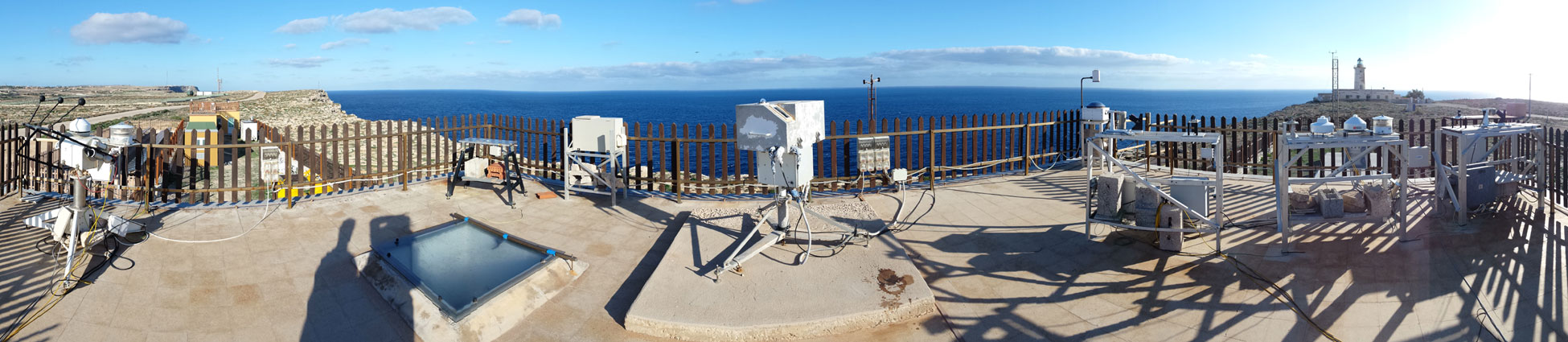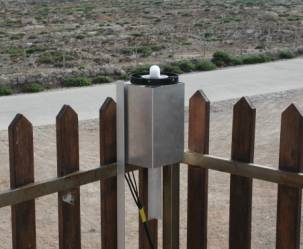# Instruments

### Diode array actinic radiation spectrometer

A Metcon DAS istrument is available at the Station for Climate Observations at Lampedusa, for measurements during campaigns. The instrument has an actinic radiation input optical system, that is coupled through an optical fiber to a Carl Zeiss spectrometer. A diode array detector measures the actinic radiation between 280 and 700 nm.

The instrument is calibrated with 1000 W NIST traceable FEL lamps, by means of the field calibrator developed at NOAA.

The actinic flux is the radiative flux of spherically integrated solar radiation through a volume of air, where the radiation is not weighted by the incidence direction (as in the irradiance definition).

The actinic flux is the proper quantity to deal with when chemical processes activated by solar radiation have to be treated. In fact, the photolysis rate of a photodissociation reaction like $AB + hv \rightarrow A + B$ can be expressed as

\begin{equation} J_{AB} \equiv \int_{\lambda_{min}}^{\lambda_{max}} F_\lambda^J \sigma_\lambda(T)\phi_\lambda(T)d\lambda \end{equation}

where $F_\lambda^J$ is the spectral actinic flux, $\sigma_\lambda$ and $\phi_\lambda$ are the absorption cross section and the quantum yield, respectively, both wavelength and temperature dependent.

One of the key reaction in tropospheric chemistry is the photolysis of ozone $(O_3)$ into $O(^1D)$ and $O_2$

\begin{equation} O_3 + hv \rightarrow O(^1D) + O_2 \end{equation}

$O(^1D)$ can react with water vapour to form the hydroxyl radical $(OH)$, which is the primary oxidizing species in the low atmosphere.

The spectral actinic fluxes have been measured at Lampedusa for several periods in 2003, 2004, 2006, and 2008 by means of the DAS spectrometer.
The measurements have been used to calculate the ozone photolysis frequency $J(O^1D)$ by means of $equation (1)$: the two integration limits are set at $\lambda_{min}=295 nm$ and $\lambda_{max}=340 nm$.
The dependence of $J(O^1D)$ on columnar ozone and aerosol optical depth at $416 nm$ has been studied and published in the paper by Casasanta G., A. di Sarra, D. Meloni, F. Monteleone, G. Pace, S. Piacentino, and D. M. Sferlazzo (2011). Large aerosol effects on ozone photolysis in the Mediterranean, Atmos. Environ. 45, 3937-3943.The actinic radiation diode array spectrometer on the roof of the Station at Lampedusa.